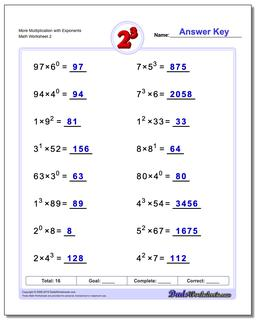# Math Worksheets: Exponents: Exponents: More Multiplication with Exponents (Second Worksheet)## More Multiplication with Exponents (Second Worksheet)

PropertyValue
DescriptionMore Multiplication with Exponents: Practice exponents worksheets mixing exponents with simple multiplication. Again, these are fantastic exercises for enhancing student comprehension of basic order of operations concepts where exponents are involved. (Second Worksheet)
Resource TypeWorksheet